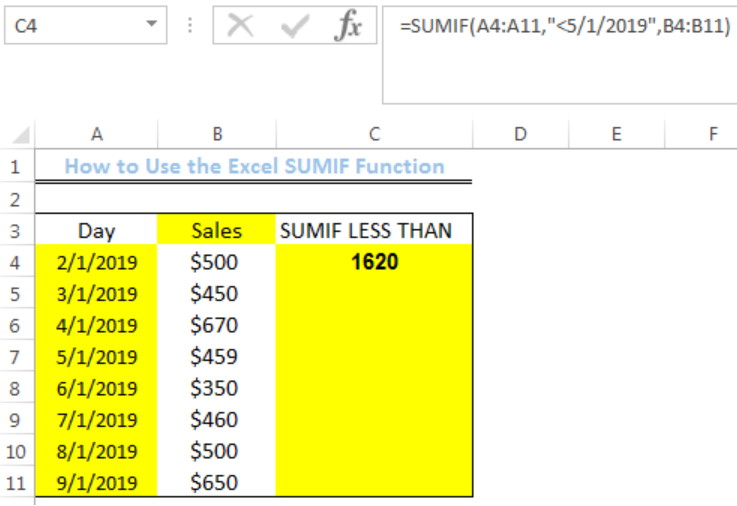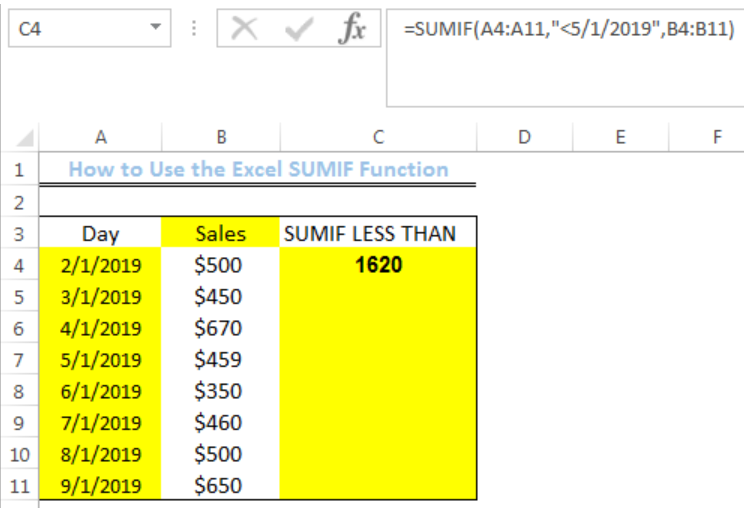Get instant live expert help with Excel or Google Sheets“My Excelchat expert helped me in less than 20 minutes, saving me what would have been 5 hours of work!”

#### Post your problem and you’ll get expert help in seconds.

Your message must be at least 40 characters
Our professional experts are available now. Your privacy is guaranteed.

# How to Use the Excel SUMIF Function

We can use the excel SUMIF function to add numbers in a range of cells based on a criteria. The steps below will walk through the process.Figure 1: How to Use the Excel SUMIF Function

## General Formula

`=SUMIF(range,criteria,[sum_range])`

## Formula

`=SUMIF(A4:A11,"<5/1/2019",B4:B11)`

## Setting up the Data

• We will set up the data by inputting the Dates of sales in Column A
• Column B will contain the sales
• Column C is where we want the formula to return the result for the SUMIF function for days before 5/1/2019Figure 2: Setting up the Data

## SUMIF Function with Criteria “<5/1/2019”

The SUMIF function checks the first range (A4:A11) for cells that have dates less than 5/1/2019. After retrieving the cells, it checks the second range (B4:B11) and sums the associated sales amount if they are in line with the criteria.

• We will click on Cell C4
• We will insert the formula below into the cell
`=SUMIF(A4:A11,"<5/1/2019",B4:B11)`
• We will press the enter keyFigure 3: Result of the SUMIF function for dates before 5/1/2019

## Notes

• The Criteria in the formula determines the cells to be added. This parameter is limited to text, number or a range and the function cannot use array constants
• Text criteria, or the criteria that include mathematical symbols, must be enclosed in double quotation marks (“”)
• The wildcard characters such as ? and * can be used in criteria. A question mark matches any ‘one’ character and an asterisk matches any ‘sequence’ of characters
• SUMIF function cannot be used with logical operators such as AND, OR, etc.

## Instant Connection to an Expert through our Excelchat Service

Most of the time, the problem you will need to solve will be more complex than a simple application of a formula or function. If you want to save hours of research and frustration, try our live Excelchat service! Our Excel Experts are available 24/7 to answer any Excel question you may have. We guarantee a connection within 30 seconds and a customized solution within 20 minutes.

### Did this post not answer your question? Get a solution from connecting with the expert.Another blog reader asked this question today on Excelchat:
Solution examplesHi, I am trying to find an average of one column 'C', where another column = a particular value, or a third column = another particular value.
Solved by T. C. in 19 minsI need a formula where s36 = 1 if a10=s7 and c10=any value?
Solved by G. J. in 13 minsHello, I need help. (Excel) Assume that columns A, C and E in a worksheet contain the names of three adults who are interested in joining the (AARP), 50 years of age and over improve the quality of their lives. Columns B, D and F contain their respective ages in whole years in the adjacent cells. Which of the following formulas should be placed in cell G1 to verify that anyof these individuals are eligible to become full members, if they are at least 50 years old? =AND(B1>49, D1>49, F1>49) =OR(B1>=50, AND(D1>=50, F1>=50)) =OR(B1>=50, D1>=50, F1>=50) =AND(B1>=50, D1>=50, F1>=50
Solved by F. Q. in 20 minsI need a long If and AND statement. If a1 is between 17-21 AND a2 is greater than 42 and a3 is greater 53 and a4 is less than the time of 15:54 then they pass, if not they fail
Solved by E. S. in 54 minsWould like to know how use the filter in excel with AND instead of OR So filtering three columns with a 0 and 1 and asking that all three show up if at least one has a number 1
Solved by B. E. in 20 mins## Subscribe to Excelchat.coAnother blog reader asked this question today on Excelchat: